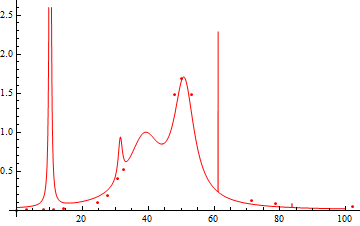# methods for interpolating a function, holomorphic in the upper halfplane

Let $n,k\colon\mathbb{R}\to\mathbb{R}$ be real functions such that function $N$ given by $N(x)=n(x)-ik(x)$ is a holomorphic function in the upper half-plane. Also I know some additional properties of these functions:
1. $n(x)=n(-x)$ if $x\in\mathbb{R}$;
2. $k(x)=-k(-x)$ if $x\in\mathbb{R}$;
3. $k(x)>0$ if $x>0$;
4. limit $\lim_{x\to\infty}(n(x)-ik(x))$ exist.

Unfortunately I don't know functions $n$ and $k$. I know only some approximate values of $n_i\simeq n(x_i)$, $k_j\simeq k(y_j)$ for finite number of points $x_i,y_j\in [a,b]\subset\mathbb{R}$. Which methods can I use in order to get approximate values of the function $N$ on the whole region $[a,b]$? Where can I read about them?

Update (example data and an experiment):

 x_j      n(x_j)
0.000  1.36364
10.204  1.32231
17.346  1.23967
18.367  1.19835
27.551  0.53719
31.632  0.53719
37.755  0.82644
42.857  0.90909
47.959  0.95041
50.000  1.32231
51.020  1.36364
53.061  2.14876
56.122  2.60331
61.224  2.47934
64.285  2.06612
67.346  1.40496
70.408  1.36364
83.673  1.94215
90.816  1.98347
102.04  1.94215

y_j     k(y_j)
0.000   0.00095
3.061   0.00288
8.163   0.00425
11.22   0.00870
14.28   0.01562
24.48   0.09638
27.55   0.18459
30.61   0.40261
32.65   0.52213
47.95   1.47684
50.00   1.68181
53.06   1.47684
71.42   0.12499
78.57   0.07931
102.0   0.04715


I've tried to fit a $n(x)$ by $\Re(f(x))$ with $f(x)=1+\sum_i\frac{c_i^2}{x-a_i-i b_i^2}$ with $c_i,a_i,b_i\in \mathbb{R}$. Here is the result of the fitting:Not a good fitting :(. This is actually why I ask this question. But note, I haven't used the data I have for k(x). Let's check, how is it approximated by $\Im(f(x))$ (with coeffitients from the previous fitting).It's better, than nothing.

• Maybe the question is not clear: your unknown function is defined on (a part of) the upper complex plane $\Im z \ge 0$, and you know only a discrete set of values of its trace at $\Im z=0$? If so, I think holomorphy plays no role Oct 14, 2010 at 8:12
• It is defined on the whole half-plane $\Im z\geq 0$. And yes, I know only something about the trace of the function. But from holomorphy I know some relations, for example this: en.wikipedia.org/wiki/Kramers%E2%80%93Kronig_relation Oct 14, 2010 at 9:44
• Well yes, if the function is in a Hardy space then the two components are Hilbert transforms of each other. What I mean is that this might not be helpful for your problem. Think of this related example: any periodic function $\sum c_ne^{inx}$ with $c_n=0$ for $n\le 0$ and $c_n$ growing at most polynomially (so it can be a distribution) is the trace of the holomorphic function $\sum c_ne^{inx-ny}$. But the two sequences of coefficients $\Re c_n$ and $\Im c_n$ are completely independent of each other Oct 14, 2010 at 10:16
• Yes, they are independent, but both can be calculated given only the real part of $f(x)=\sum c_n e^{inx}$ (if $c_n\to 0$ fast enough): $c_n=\lim_{N\to\infty} \int_{-N}^{N} \Re(f(x))e^{inx}/N dx$. I understand, that function cannot be determined by its values in the finite number of points. Nevertheless we are measuring, for example, temperature and then we are interpolating it between the points. Ok, we use some assumptions on the dependence of temperature on time. But it works. Oct 14, 2010 at 12:13
• Is the upper bound $M_1$ for $n(x)$ known, or just that it exists? The latter follows from continuity and the fact it's approaching $1$ at $\pm \infty$. Oct 17, 2010 at 13:21

If I understand correctly, we can make a change of variables from $z$ in the upper half plane to $\zeta$ in the unit disk. Then the function $N(\zeta)$ is continuous on the unit circle $\zeta = \exp(i \theta)$ and has a unique harmonic extension to the unit disk. If $N$ is a favorable function, then this harmonic extension is complex analytic. A function in this class is one with a Taylor series in $\zeta$ with a radius of convergence at least 1. Or, it is a Fourier series in $\theta$ which uses only terms $\exp(i k \theta)$ with $k \ge 0$, and whose coefficients satisfy a convergence condition.
In your question, in addition, the real and imaginary parts of $N(\zeta)$ satisfy certain inequalities, and they are supposed to be as close as possible to certain data points. Let's assume a least-squares error model for these data points. If you put all of this information together, the result is that the Fourier coefficients $a_k$ lie in a certain convex region, and you want to minimize a certain quadratic objective function. Thus, your question is a convex programming problem. You can make this into a practical computational convex programming problem by assuming that $k \le 100$ (say) and also discretizing the unit circle on which $\zeta$ lies. Or, there are also numerical methods to impose your constraints (1) and (2) without discretizing the unit circle, but they make the convex programming problem more complicated.
• Concretely, Greg's suggestion amounts to fitting your original function $N(x)$ by $\sum_{k\ge 0} a_k \left(\frac{i-z}{i+z}\right)^k$. I might try doing this naively first, and hoping that the additional convex constraints are satisfied automatically. Oct 17, 2010 at 5:32